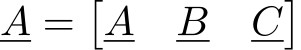# 数学环境下行内向量的基线对齐问题

\documentclass{standalone}
\usepackage{amsmath}
\begin{document}
$\underline{A}=\begin{bmatrix} \underline{A} & \underline{B} & \underline{C} \end{bmatrix}$
\end{document}\documentclass{standalone}
\usepackage{amsmath}
\newcommand*{\rowvector}
{%
\begingroup%
\everymath{\displaystyle}%
\begin{array}[t]{@{}*#1 c@{}}
#2
\end{array}%
\endgroup%
}
\newcommand*{\browvector}{\left[\rowvector{#1}{#2}\right]}
\begin{document}
$\underline{A}=\browvector{3}{\underline{A} & \underline{B} & \underline{C}}$
\end{document}